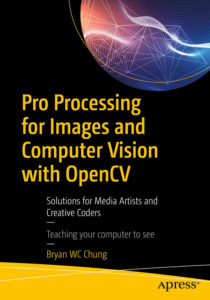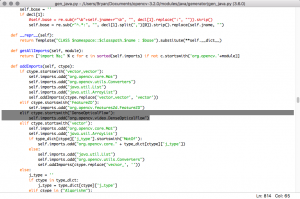OpenCV 3.4.2 Java Build

After the release of OpenCV 3.4.2, I have prepared the pre-built version of the Java libraries for OSX, Ubuntu, and Windows 8.1 platforms (64 bits).  In this release, there is more extensive support for the Java binding. I also packaged the library as the Processing library – CVImage. Please refer to latest book for details. In addition to the optflow contributed library, it also includes additional contributed libraries, such as bgsegm, face, and tracking.

CVImage for OpenCV 3.4.2

CVImage and PixelFlow in Processing

This is a quick demonstration of using the CVImage library, from the book, Pro Processing for Images and Computer Vision with OpenCV, and the PixelFlow library from Thomas Diewald.

Here is the video documentation.

The full source code is below with one additional class.

Main Processing sketch

 import cvimage.*; import processing.video.*; import com.thomasdiewald.pixelflow.java.DwPixelFlow; import com.thomasdiewald.pixelflow.java.fluid.DwFluid2D; import org.opencv.core.*; import org.opencv.objdetect.CascadeClassifier; import org.opencv.objdetect.Objdetect;   // Face detection size final int W = 320, H = 180; Capture cap; CVImage img; CascadeClassifier face; float ratio; DwFluid2D fluid; PGraphics2D pg_fluid; MyFluidData fluidFunc;   void settings() { size(1280, 720, P2D); }   void setup() { background(0); System.loadLibrary(Core.NATIVE_LIBRARY_NAME); println(Core.VERSION); cap = new Capture(this, width, height); cap.start(); img = new CVImage(W, H); face = new CascadeClassifier(dataPath("haarcascade_frontalface_default.xml")); ratio = float(width)/W;   DwPixelFlow context = new DwPixelFlow(this); context.print(); context.printGL(); fluid = new DwFluid2D(context, width, height, 1); fluid.param.dissipation_velocity = 0.60f; fluid.param.dissipation_density = 0.99f; fluid.param.dissipation_temperature = 1.0f; fluid.param.vorticity = 0.001f;   fluidFunc = new MyFluidData(); fluid.addCallback_FluiData(fluidFunc); pg_fluid = (PGraphics2D) createGraphics(width, height, P2D); pg_fluid.smooth(4); }   void draw() { if (!cap.available()) return; background(0); cap.read(); cap.updatePixels();   img.copy(cap, 0, 0, cap.width, cap.height, 0, 0, img.width, img.height); img.copyTo();   Mat grey = img.getGrey(); MatOfRect faces = new MatOfRect();   face.detectMultiScale(grey, faces, 1.15, 3, Objdetect.CASCADE_SCALE_IMAGE, new Size(60, 60), new Size(200, 200)); Rect [] facesArr = faces.toArray(); if (facesArr.length > 0) { fluidFunc.findFace(true); } else { fluidFunc.findFace(false); } for (Rect r : facesArr) { float cx = r.x + r.width/2.0; float cy = r.y + r.height/2.0; fluidFunc.setPos(new PVector(cx*ratio, cy*ratio)); } fluid.update(); pg_fluid.beginDraw(); pg_fluid.background(0); pg_fluid.image(cap, 0, 0); pg_fluid.endDraw(); fluid.renderFluidTextures(pg_fluid, 0); image(pg_fluid, 0, 0); pushStyle(); noStroke(); fill(0); text(nf(round(frameRate), 2, 0), 10, 20); popStyle(); grey.release(); faces.release(); }

The class definition of MyFluidData

 private class MyFluidData implements DwFluid2D.FluidData { float intensity; float radius; float temperature; color c; boolean first; boolean face; PVector pos; PVector last;   public MyFluidData() { super(); intensity = 1.0f; radius = 25.0f; temperature = 5.0f; c = color(255, 255, 255); first = true; pos = new PVector(0, 0); last = new PVector(0, 0); face = false; }   public void findFace(boolean f) { face = f; }   public void setPos(PVector p) { if (first) { pos.x = p.x; pos.y = p.y; last.x = pos.x; last.y = pos.y; first = false; } else { last.x = pos.x; last.y = pos.y; pos.x = p.x; pos.y = p.y; } }   @Override public void update(DwFluid2D f) {   if (face) { float px = pos.x; float py = height - pos.y; float vx = (pos.x - last.x) * 10.0f; float vy = (pos.y - last.y) * -10.0f; c = color(random(100, 255), random(100, 255), random(50, 100)); f.addVelocity(px, py, radius, vx, vy); f.addDensity (px, py, radius, red(c)/255, green(c)/255, blue(c)/255, intensity); f.addTemperature(px, py, radius, temperature); } } }

OpenCV 3.3 Java Build

The new release of OpenCV 3.3 is out now. I again prepare the Java build for the CVImage Processing library use. It also includes the optflow extra module for motion history applications. Here is the list of the 3 OpenCV releases.

The book Pro Processing for Images and Computer Vision with OpenCV will be released soon. It will include the detailed build instructions in multiple platforms.OpenCV 3.2 Java Build

In preparing for the forthcoming book in Processing and OpenCV, I have tried to build the Java binding in OpenCV 3.2. It worked easily for the basic components. Nevertheless, when I included the contribution moduleoptflow, it failed. After a number of attempts in various platforms, I found it was due to the gen_java.py script in folder opencv-3.2.0/modules/java/generator. I tried to add back the import details for the class DenseOpticalFlow. It worked again. Here is what I patch in the gen_java.py script.For those who do not want to build it yourselves, you can download a pre-built version of the OpenCV 3.2 Java library. You can use it with Processing immediately. I have tested it with the current Processing at 3.3. It contains the following files for various platforms in 64 bit:

• libopencv_java320.dylib
• libopencv_java320.so
• opencv_java320.dll
• opencv-320.jar

Enjoy and happy coding.

OpenCV and Processing 1

This is the beginning of a series of posts related to using OpenCV and Processing in the Linux environment. I built the OpenCV 3.0.0 rc1 in Ubuntu. By putting the 2 files, libopencv_java300.so and opencv-300.jar in the code folder of a sketch, I can use the alpha version 3.0a7 of Processing and the official Java binding of OpenCV together to prepare for the examples of a newly proposed book in image processing and computer vision. I do not use the OpenCV for Processing library by Greg Borenstein, in order to reveal the underlying working mechanism of OpenCV for learning purpose.